# Parallel Lines What do you mean parallel Parallel

• Slides: 37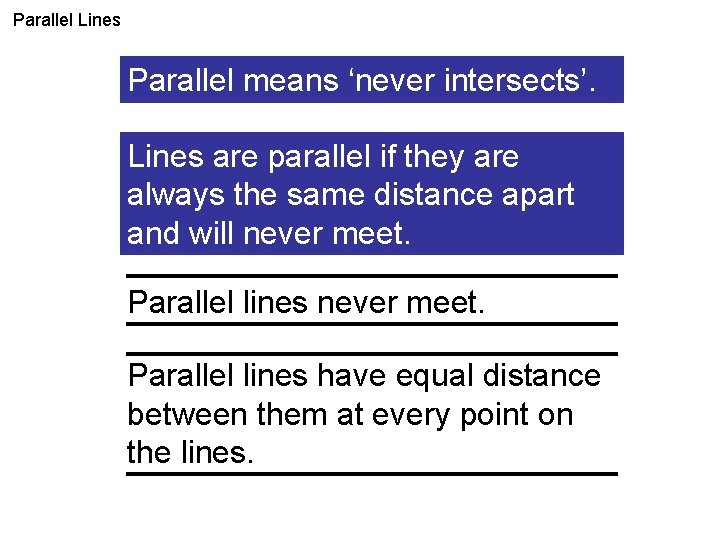Parallel Lines What do you mean parallel? Parallel means ‘neverbyintersects’. Lines are parallel if they are always the same distance apart and will never meet. Parallel lines have equal distance between them at every point on the lines.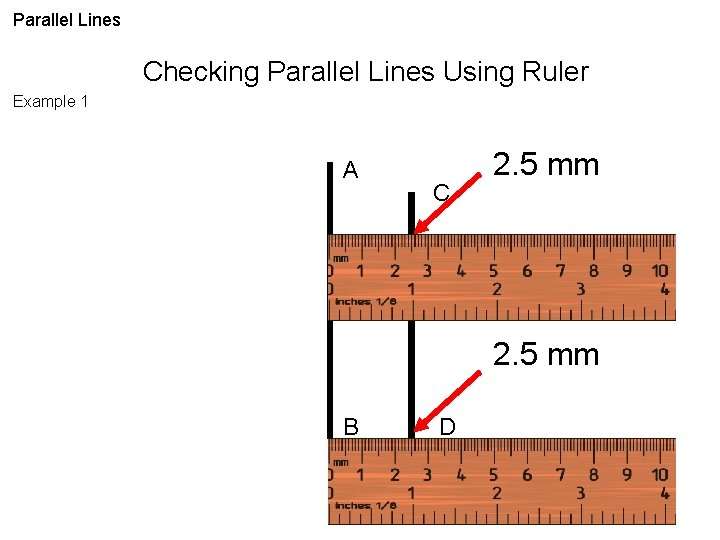Parallel Lines Checking Parallel Lines Using Ruler Example 1 A C 2. 5 mm B DParallel Lines Checking Parallel Lines Using Ruler Example 1 A DC is parallel to AB C 2. 5 mm DC ll AB 2. 5 mm B D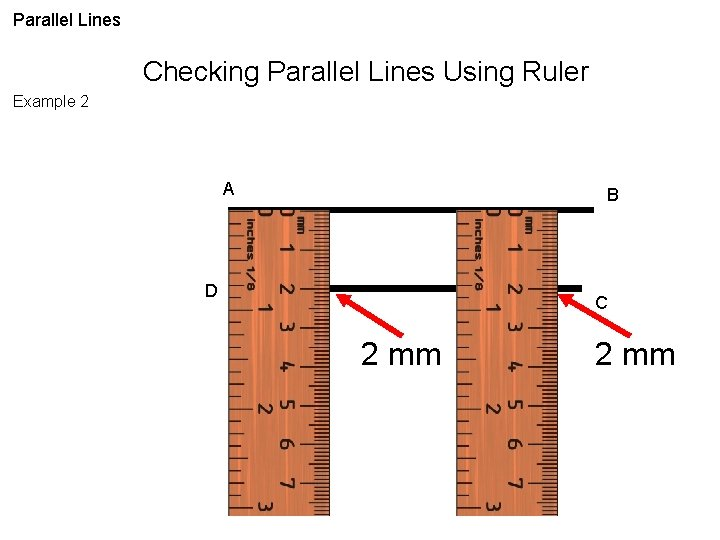Parallel Lines Checking Parallel Lines Using Ruler Example 2 A B D C 2 mm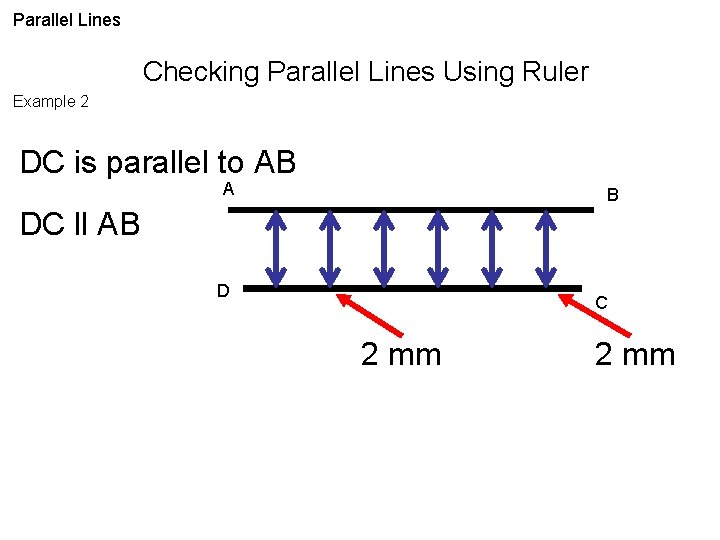Parallel Lines Checking Parallel Lines Using Ruler Example 2 DC is parallel to AB A B DC ll AB D C 2 mm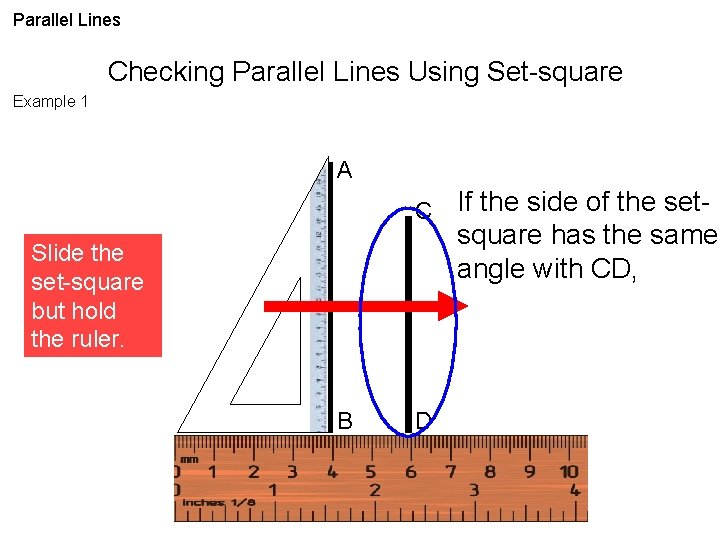Parallel Lines Checking Parallel Lines Using Set-square Example 1 A C Slide the set-square but hold the ruler. B D If the side of the setsquare has the same angle with CD,Parallel Lines Checking Parallel Lines Using Set-square Example 1 A C If the side of the setsquare has the same angle with CD, DC is parallel to AB. DC ll AB B DParallel Lines Checking Parallel Lines Using Set-square Example 2 A If the side of the setsquare has the same angle with CD, C Slide the set-square but hold the ruler. B DParallel Lines Checking Parallel Lines Using Set-square Example 2 A If the side of the setsquare has the same angle with CD, C DC is parallel to AB. DC ll AB B DParallel Lines Checking Parallel Lines Example 1 CL is parallel to AF Is AF II CL? A C F L YES CL ll AF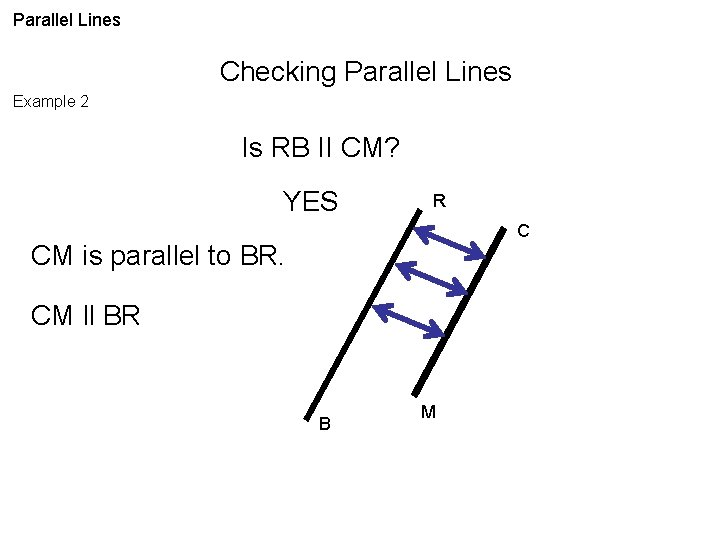Parallel Lines Checking Parallel Lines Example 2 Is RB II CM? YES R C CM is parallel to BR. CM ll BR B MParallel Lines Checking Parallel Lines Example 3 Is EF II ON? EF is parallel to ON EF ll ON YES E F O N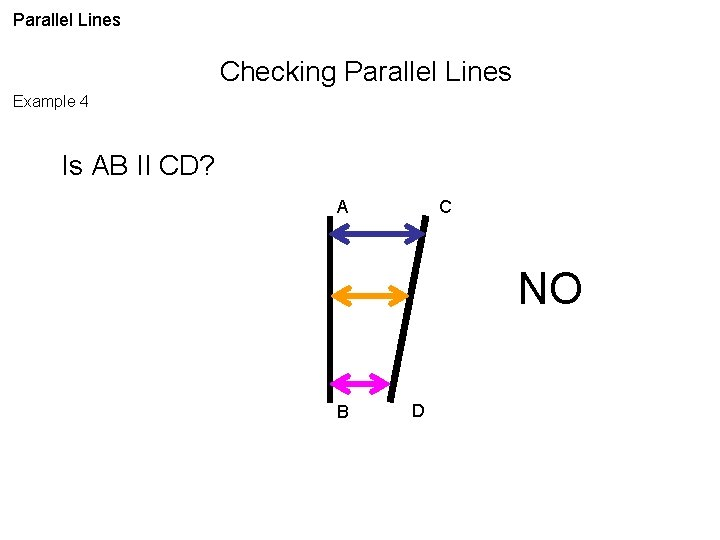Parallel Lines Checking Parallel Lines Example 4 Is AB II CD? A C NO B D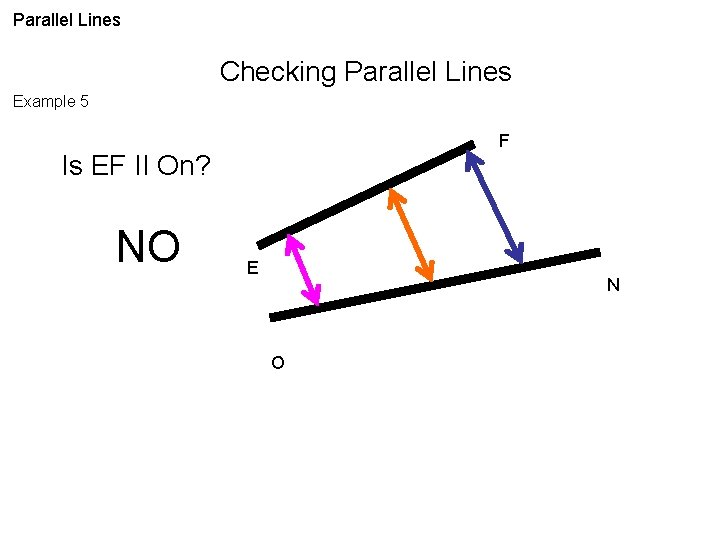Parallel Lines Checking Parallel Lines Example 5 F Is EF II On? NO E N O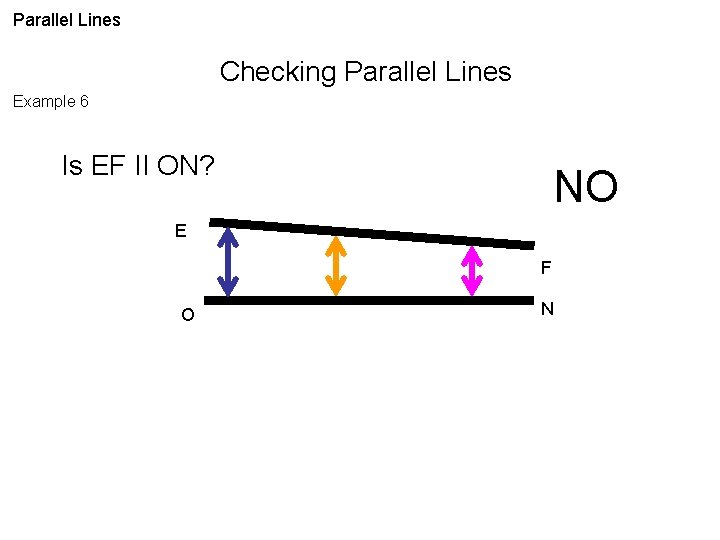Parallel Lines Checking Parallel Lines Example 6 Is EF II ON? NO E F O N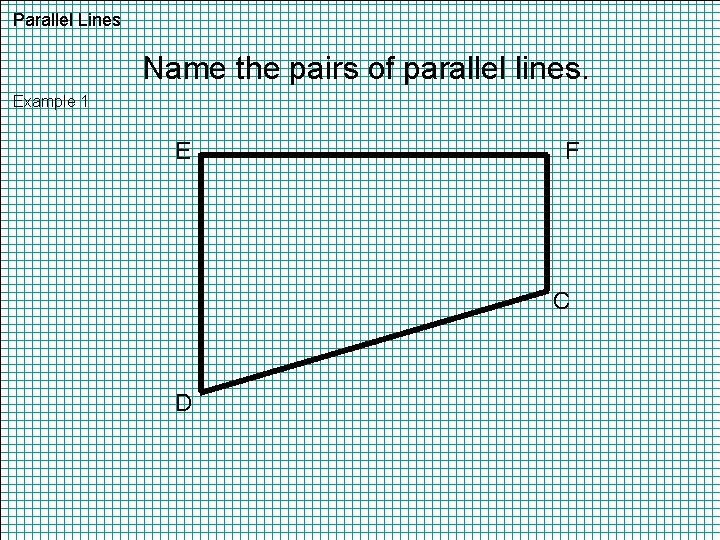Parallel Lines Name the pairs of parallel lines. Example 1 E F C D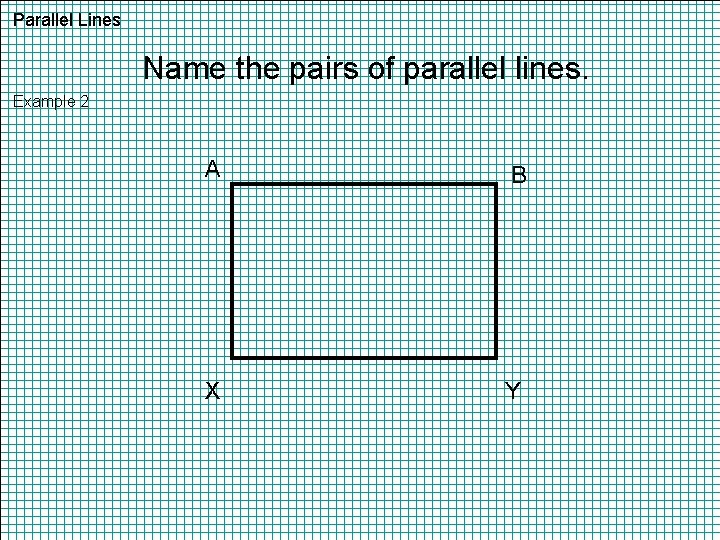Parallel Lines Name the pairs of parallel lines. Example 2 A B X YParallel Lines Name the pairs of parallel lines. Example 3 E D C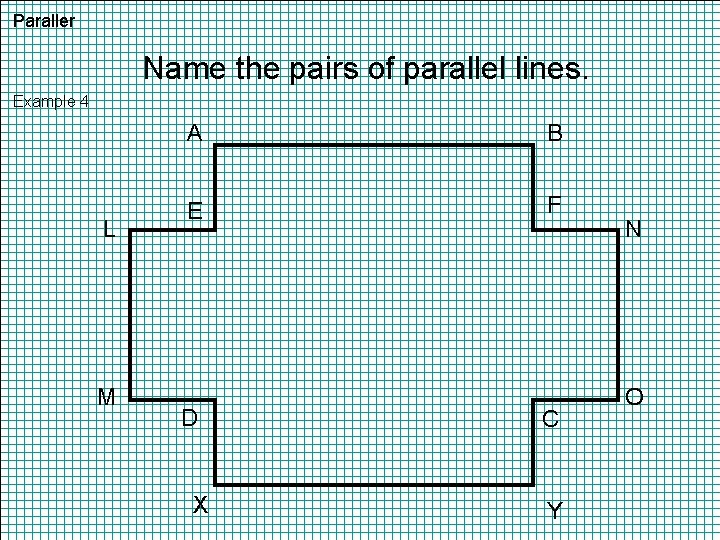Paraller Name the pairs of parallel lines. Example 4 L M A B E F D X C Y N O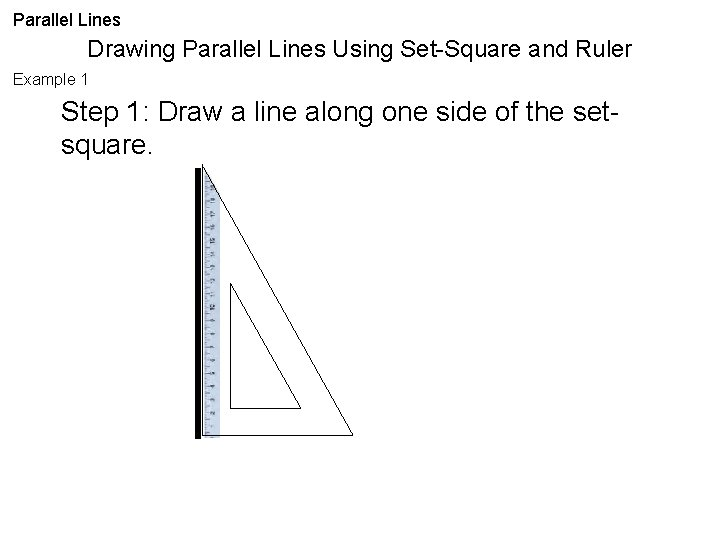Parallel Lines Drawing Parallel Lines Using Set-Square and Ruler Example 1 Step 1: Draw a line along one side of the setsquare.Parallel Lines Drawing Parallel Lines Using Set-Square and Ruler Example 1 Step 2: Place a ruler along the other side of the set-square. Then slide the set-square.Parallel Lines Drawing Parallel Lines Using Set-Square and Ruler Example 1 Step 3: Draw a line along the side of the setsquare.Parallel Lines Drawing Parallel Lines Using Set-Square and Ruler Example 2 Step 1: Draw a line along one side of the setsquare.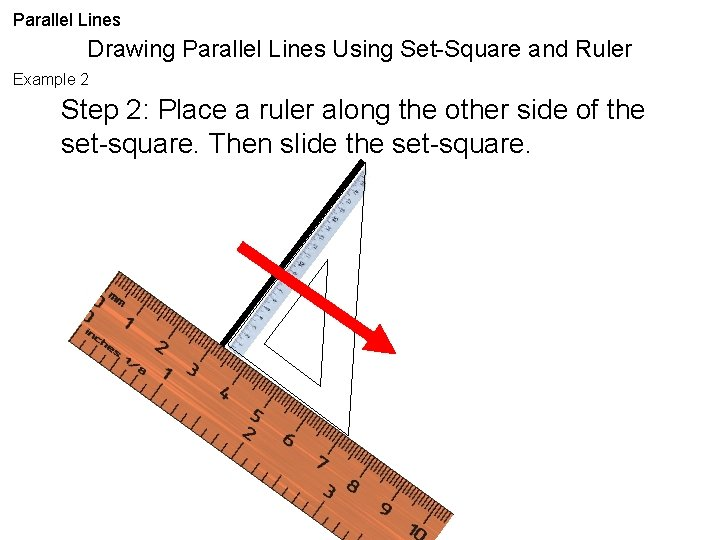Parallel Lines Drawing Parallel Lines Using Set-Square and Ruler Example 2 Step 2: Place a ruler along the other side of the set-square. Then slide the set-square.Parallel Lines Drawing Parallel Lines Using Set-Square and Ruler Example 2 Step 3: Draw a line along the side of the setsquare.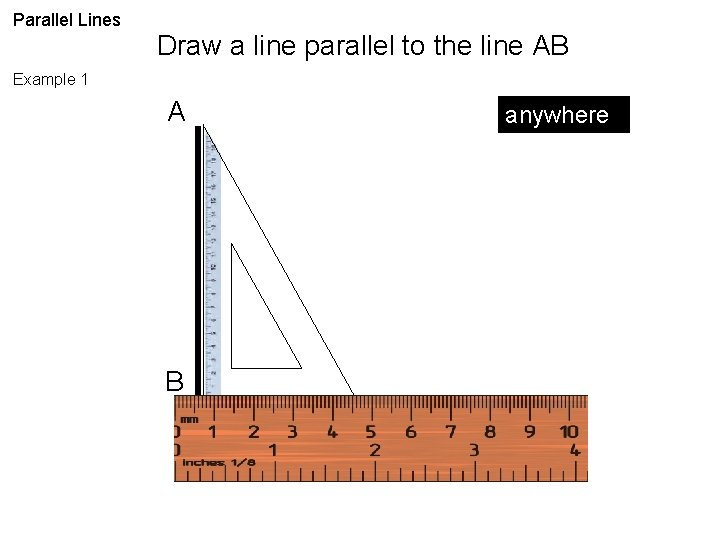Parallel Lines Draw a line parallel to the line AB Example 1 A B anywhere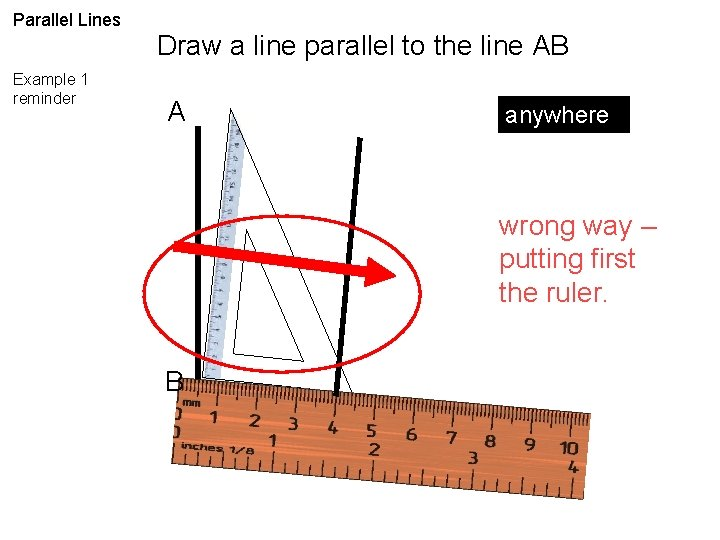Parallel Lines Example 1 reminder Draw a line parallel to the line AB A anywhere wrong way – putting first the ruler. B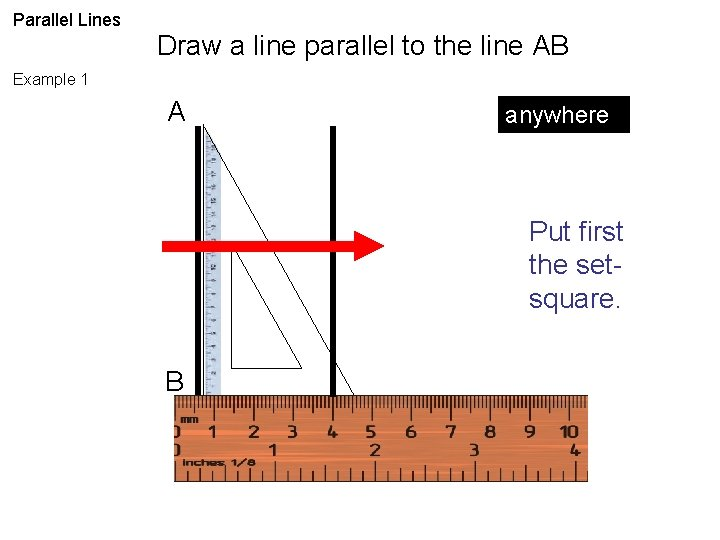Parallel Lines Draw a line parallel to the line AB Example 1 A anywhere Put first the setsquare. B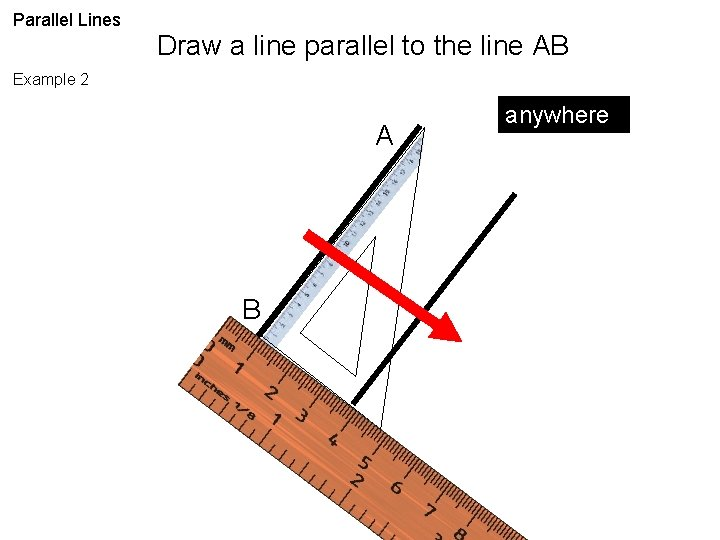Parallel Lines Draw a line parallel to the line AB Example 2 A B anywhere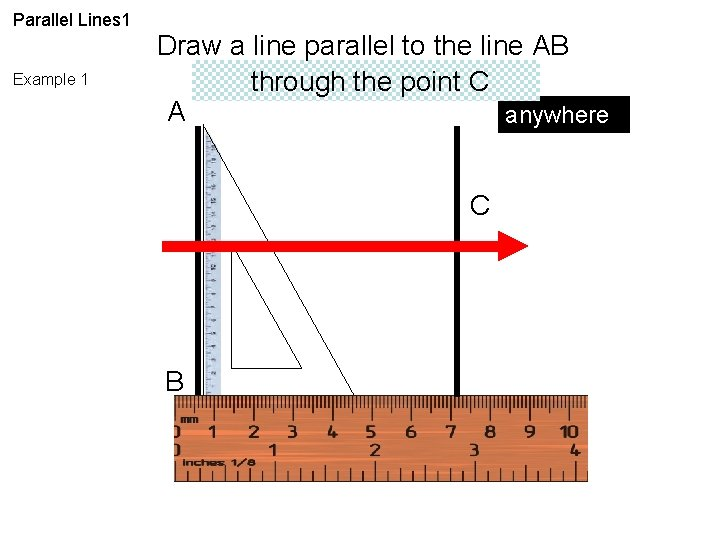Parallel Lines 1 Example 1 Draw a line parallel to the line AB through the point C A anywhere . C B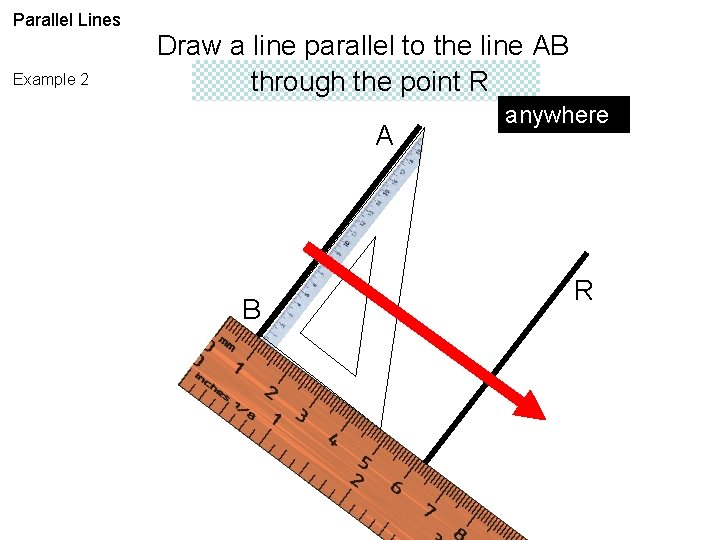Parallel Lines Example 2 Draw a line parallel to the line AB through the point R A B anywhere . RParallel Lines Example 3 Draw a line parallel to the line AB through the point X anywhere B A . XPerpendicular Reminders Align properly the line and the side of the set-square. A B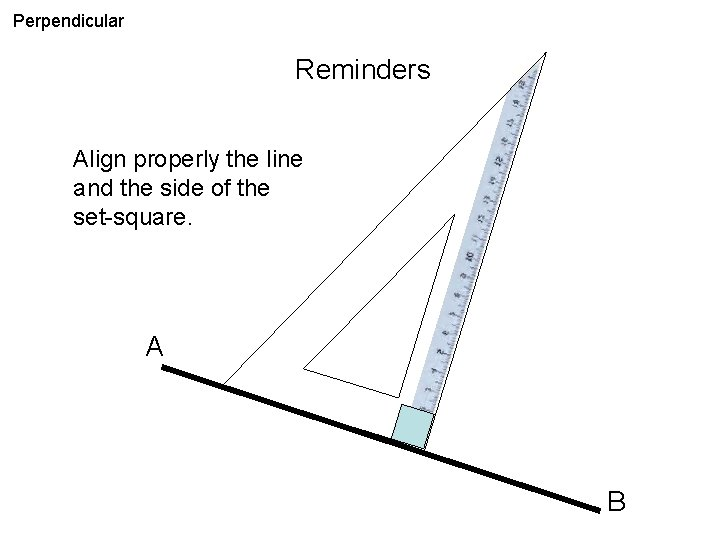Perpendicular Reminders Align properly the line and the side of the set-square. A BPerpendicular Reminders Align properly the line and the side of the set-square. A B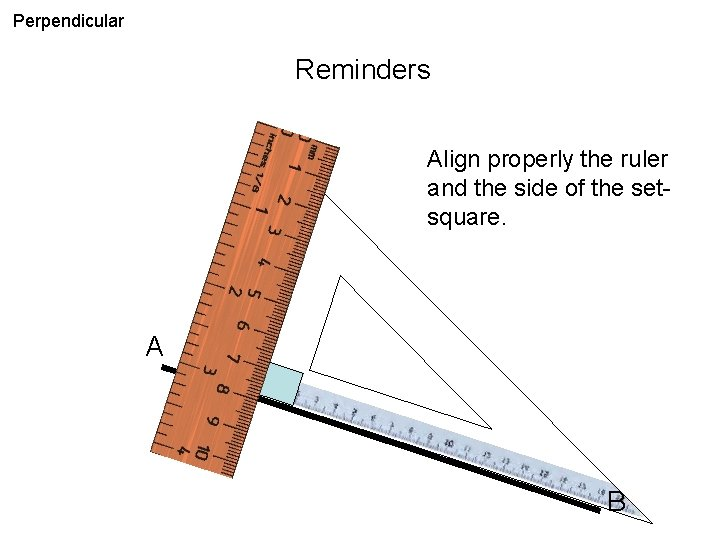Perpendicular Reminders Align properly the ruler and the side of the setsquare. A BPerpendicular Reminders Protractor can be used also as a guide. A B